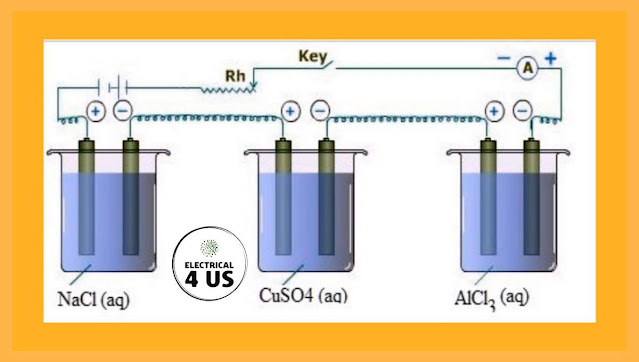## Posts

Showing posts with the label Electrolysis

### What is Faraday's law of Electrolysis?Faraday's law of electrolysis The relationship between the amount of electric charge passing through an electrolyte and the amount of substance deposited at the electrode was introduced by Faraday in 1834 as the laws of electrolysis. 1. first law The mass of the substance deposited or liberated at any electrode is directly proportional to the amount of charge passed" Let this proportionality be called w = zq.  can be made in equality by Where z is the proportionality constant called the electrochemical equivalent.  It is the mass of matter in grams that is deposited or released by passing a coulomb of charge. 2. Second Law The mass of a substance that is deposited or liberated at an electrode when a certain amount of charge is passed is directly proportional to its chemical equivalent mass". that is w a E where w is the mass of the substance in grams while E is its chemical equivalent weight per gram equivalent. This law can be explained as follows. Consider three reactio